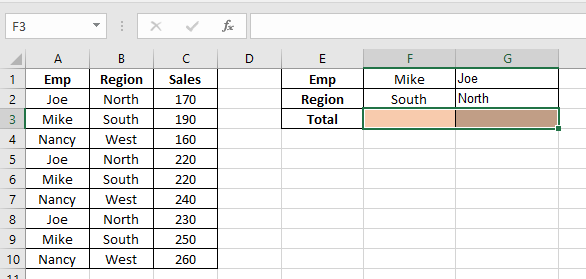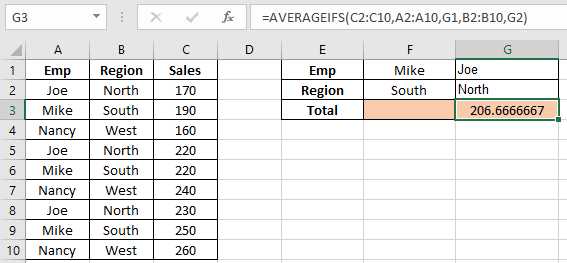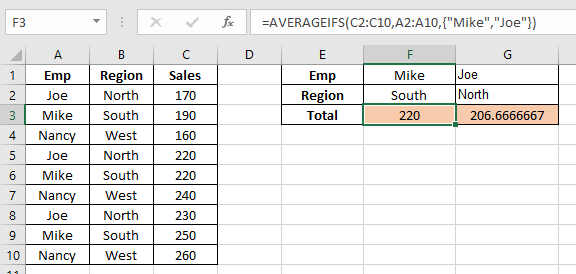# How to use the Excel AVERAGEIFS function

In this article, we will learn about how to use AVERAGEIFS functions in Excel.

Excel AVERAGEIFS function is used to find the average of the array based on multiple criteria or condition.
Syntax:

=AVERAGEIFS (average_range, range1, criteria1, [range2,criteria2])

Let’s understand this function using it an example.Here we need to find the average of the Sales having name “Joe” & region “North”

Use the formula to get the average

=AVERAGEIFS(C2:C10, A2:A10, G1, B2:B10, G2)

C2:C10 : Range where the average needs to be calculated
A2:A10 : Range where Name “Joe” criteria occur
G1 : Match value
B2:B10 : Range where Region “North” criteria occur
G2 : Match value206.67 is Joe’s North region sales average

Now we need to find the average Sales of combined joe’s and Mike’s

Use the formula

=AVERAGEIFS(C2:C10,A2:A10,{"Mike","Joe"})As you can see the average for the data is here.

Hope you learnt how to use AVERAGEIFS function from the above article. Explore more articles here on mathematical formulation. Please feel free to state your queries in the comment box below.

Related Articles:

How to Calculate Mean in Excel

Ignore zero in the Average of numbers

Calculate Weighted Average

Average Difference between lists

Popular Articles:

How to use the VLOOKUP Function in Excel

How to use the COUNTIF function in Excel 2016

How to Use SUMIF Function in Excel

Terms and Conditions of use

The applications/code on this site are distributed as is and without warranties or liability. In no event shall the owner of the copyrights, or the authors of the applications/code be liable for any loss of profit, any problems or any damage resulting from the use or evaluation of the applications/code.